# 093011 Straight Lines and Hough Computer Vision CS

• Slides: 3409/30/11 Straight Lines and Hough Computer Vision CS 143, Brown James Hays Many slides from Derek Hoiem, Lana Lazebnik, Steve Seitz, David Forsyth, David Lowe, Fei-Fei Li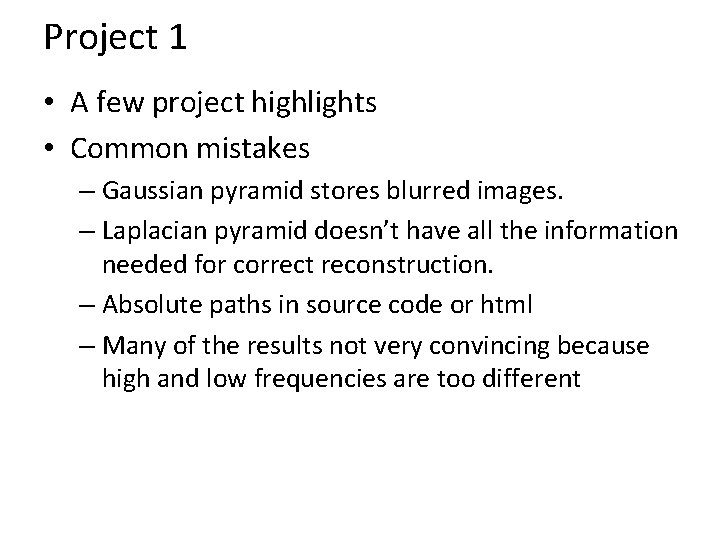Project 1 • A few project highlights • Common mistakes – Gaussian pyramid stores blurred images. – Laplacian pyramid doesn’t have all the information needed for correct reconstruction. – Absolute paths in source code or html – Many of the results not very convincing because high and low frequencies are too differentProject 2 • Questions?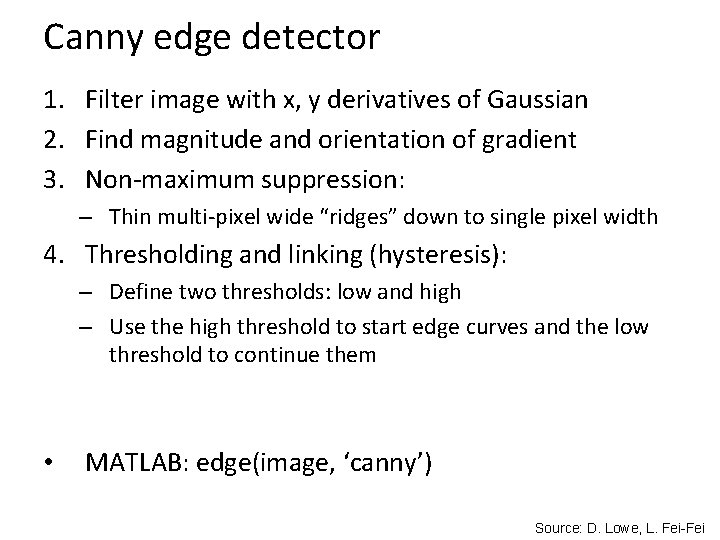Canny edge detector 1. Filter image with x, y derivatives of Gaussian 2. Find magnitude and orientation of gradient 3. Non-maximum suppression: – Thin multi-pixel wide “ridges” down to single pixel width 4. Thresholding and linking (hysteresis): – Define two thresholds: low and high – Use the high threshold to start edge curves and the low threshold to continue them • MATLAB: edge(image, ‘canny’) Source: D. Lowe, L. Fei-FeiFinding straight lines • One solution: try many possible lines and see how many points each line passes through • Hough transform provides a fast way to do thisHough transform • An early type of voting scheme • General outline: • Discretize parameter space into bins • For each feature point in the image, put a vote in every bin in the parameter space that could have generated this point • Find bins that have the most votes Image space Hough parameter space P. V. C. Hough, Machine Analysis of Bubble Chamber Pictures, Proc. Int. Conf. High Energy Accelerators and Instrumentation, 1959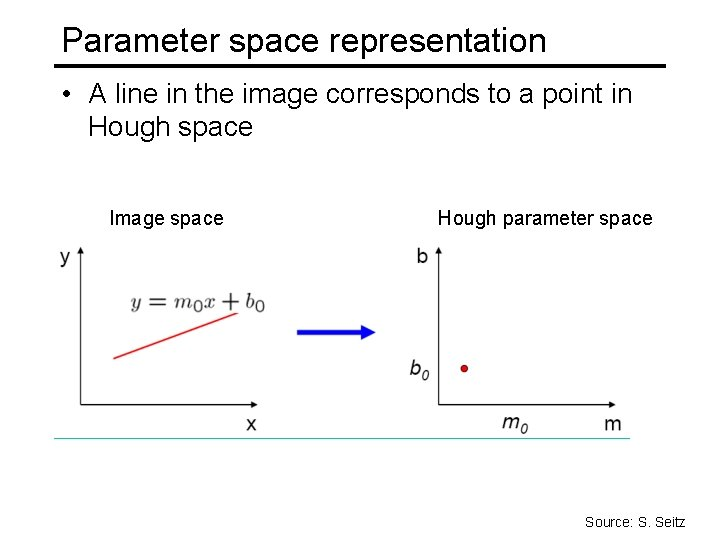Parameter space representation • A line in the image corresponds to a point in Hough space Image space Hough parameter space Source: S. SeitzParameter space representation • What does a point (x 0, y 0) in the image space map to in the Hough space? Image space Hough parameter space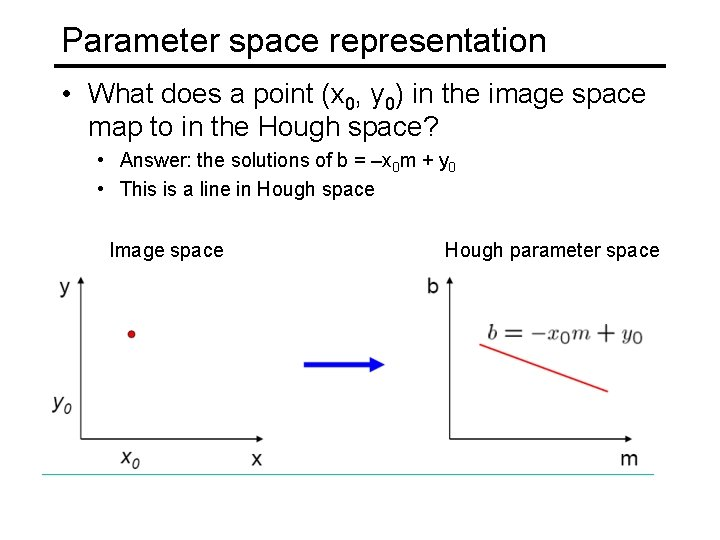Parameter space representation • What does a point (x 0, y 0) in the image space map to in the Hough space? • Answer: the solutions of b = –x 0 m + y 0 • This is a line in Hough space Image space Hough parameter space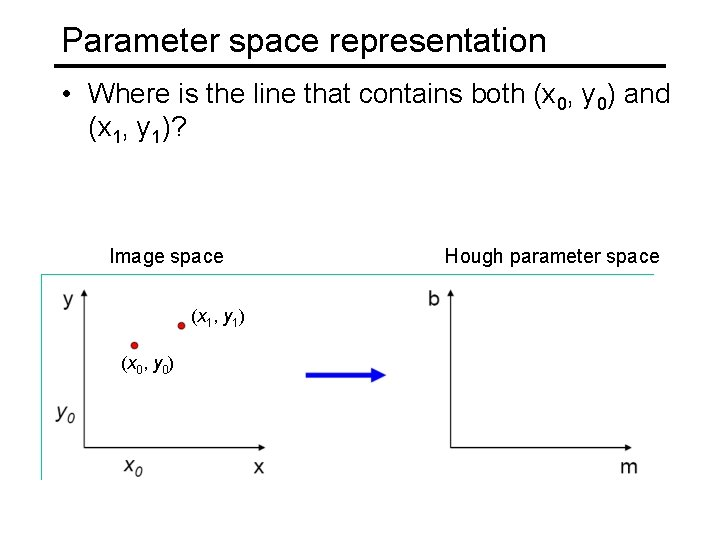Parameter space representation • Where is the line that contains both (x 0, y 0) and (x 1, y 1)? Image space Hough parameter space (x 1, y 1) (x 0, y 0) b = –x 1 m + y 1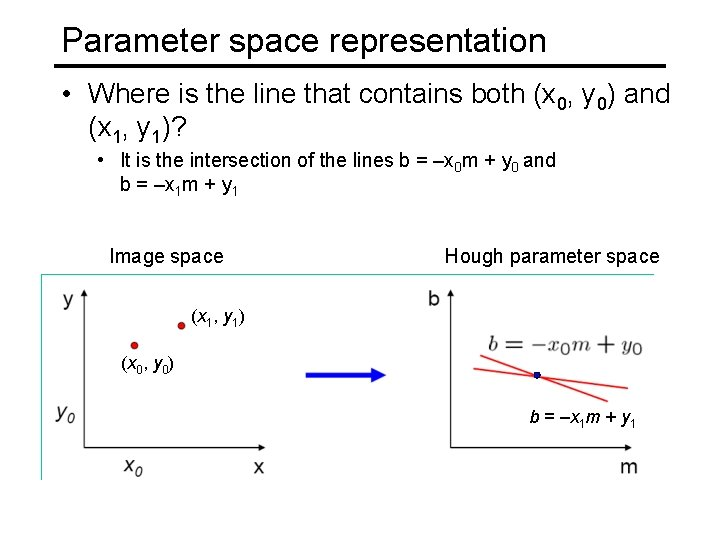Parameter space representation • Where is the line that contains both (x 0, y 0) and (x 1, y 1)? • It is the intersection of the lines b = –x 0 m + y 0 and b = –x 1 m + y 1 Image space Hough parameter space (x 1, y 1) (x 0, y 0) b = –x 1 m + y 1Parameter space representation • Problems with the (m, b) space: • Unbounded parameter domain • Vertical lines require infinite mParameter space representation • Problems with the (m, b) space: • Unbounded parameter domain • Vertical lines require infinite m • Alternative: polar representation Each point will add a sinusoid in the ( , ) parameter spaceAlgorithm outline • • • Initialize accumulator H to all zeros For each edge point (x, y) ρ in the image For θ = 0 to 180 ρ = x cos θ + y sin θ θ H(θ, ρ) = H(θ, ρ) + 1 end Find the value(s) of (θ, ρ) where H(θ, ρ) is a local maximum • The detected line in the image is given by ρ = x cos θ + y sin θ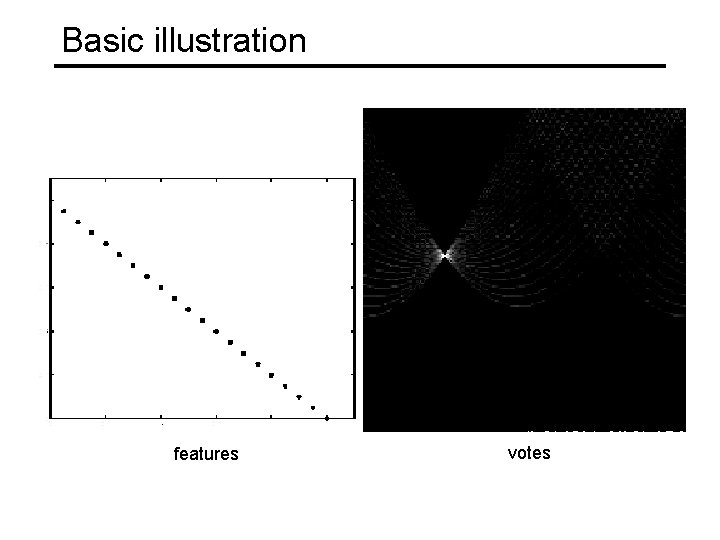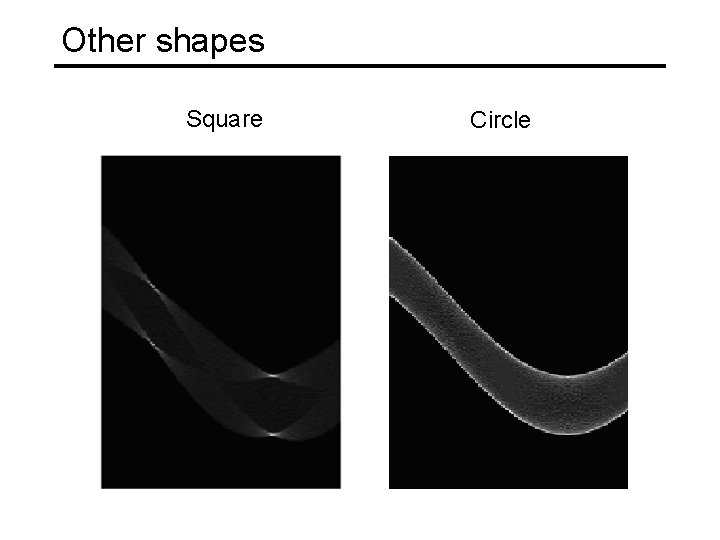Other shapes Square CircleSeveral linesA more complicated image http: //ostatic. com/files/images/ss_hough. jpg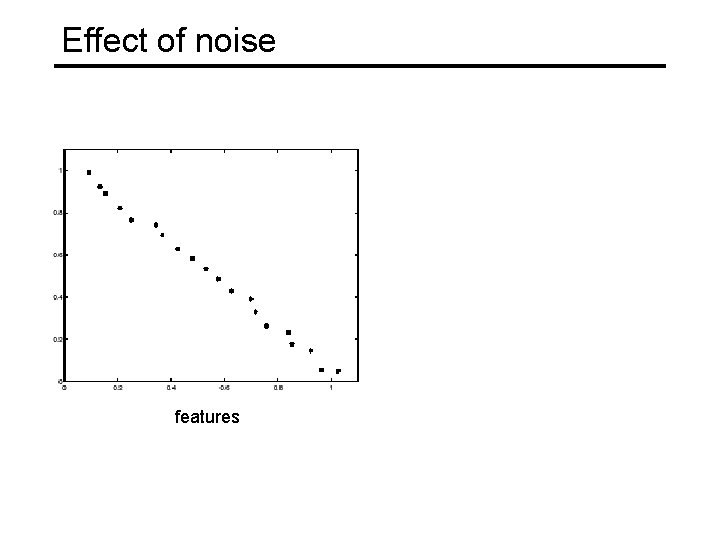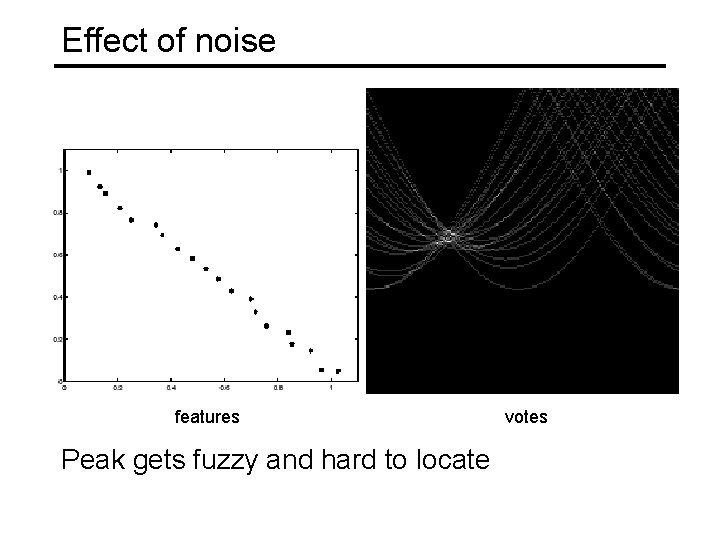Effect of noise features Peak gets fuzzy and hard to locate votes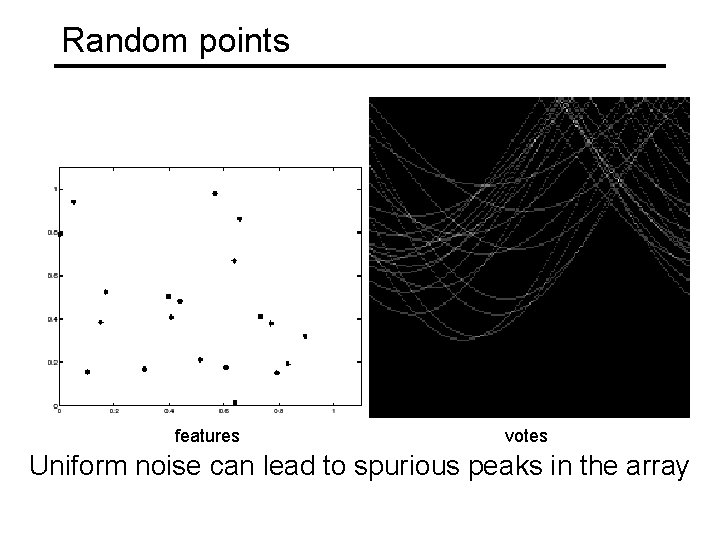Random points features votes Uniform noise can lead to spurious peaks in the array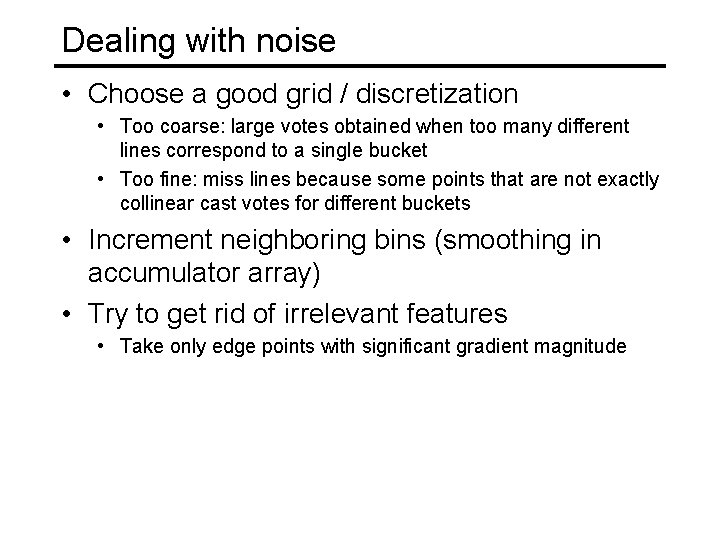Dealing with noise • Choose a good grid / discretization • Too coarse: large votes obtained when too many different lines correspond to a single bucket • Too fine: miss lines because some points that are not exactly collinear cast votes for different buckets • Increment neighboring bins (smoothing in accumulator array) • Try to get rid of irrelevant features • Take only edge points with significant gradient magnitude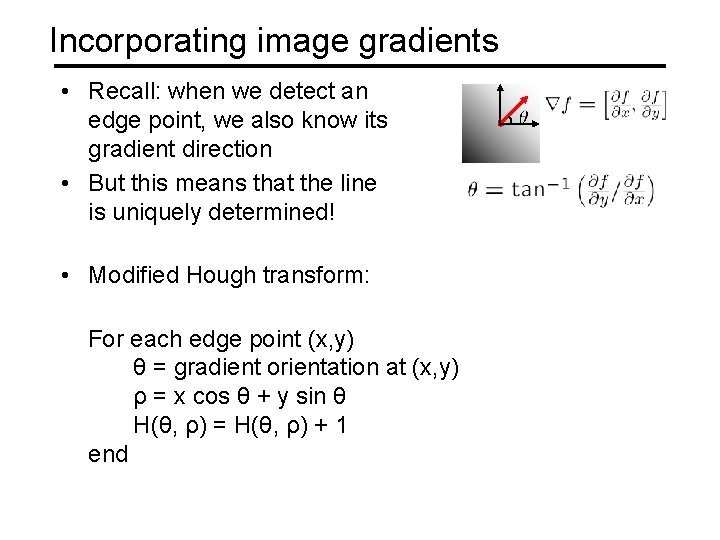Incorporating image gradients • Recall: when we detect an edge point, we also know its gradient direction • But this means that the line is uniquely determined! • Modified Hough transform: For each edge point (x, y) θ = gradient orientation at (x, y) ρ = x cos θ + y sin θ H(θ, ρ) = H(θ, ρ) + 1 end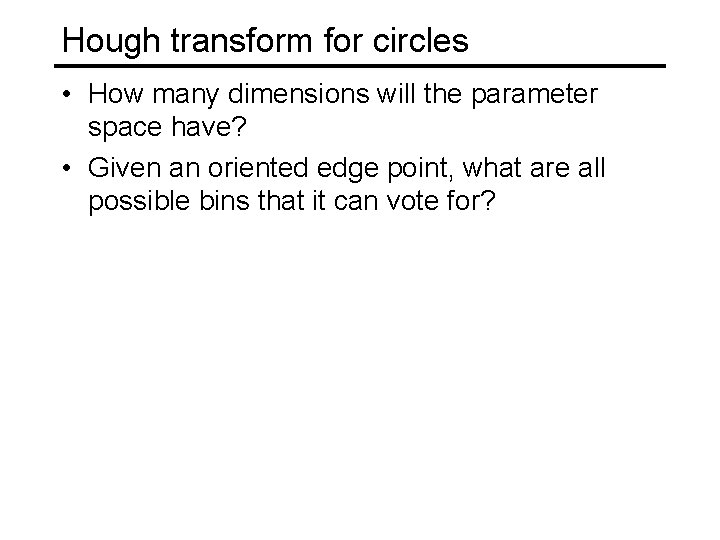Hough transform for circles • How many dimensions will the parameter space have? • Given an oriented edge point, what are all possible bins that it can vote for?Hough transform for circles image space Hough parameter space r y (x, y) x x yHough transform for circles • Conceptually equivalent procedure: for each (x, y, r), draw the corresponding circle in the image and compute its “support” r x y Is this more or less efficient than voting with features?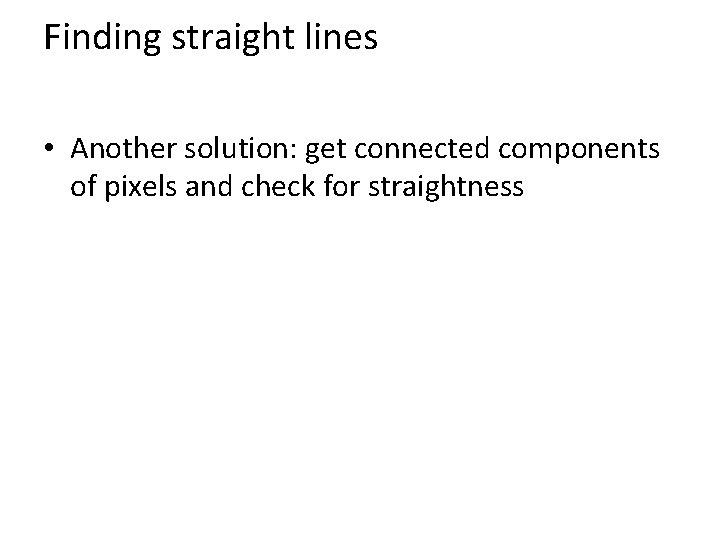Finding straight lines • Another solution: get connected components of pixels and check for straightness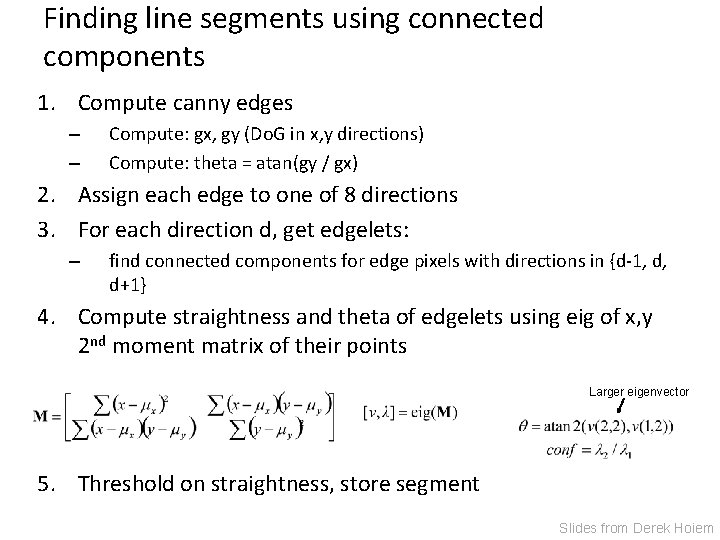Finding line segments using connected components 1. Compute canny edges – – Compute: gx, gy (Do. G in x, y directions) Compute: theta = atan(gy / gx) 2. Assign each edge to one of 8 directions 3. For each direction d, get edgelets: – find connected components for edge pixels with directions in {d-1, d, d+1} 4. Compute straightness and theta of edgelets using eig of x, y 2 nd moment matrix of their points Larger eigenvector 5. Threshold on straightness, store segment Slides from Derek Hoiem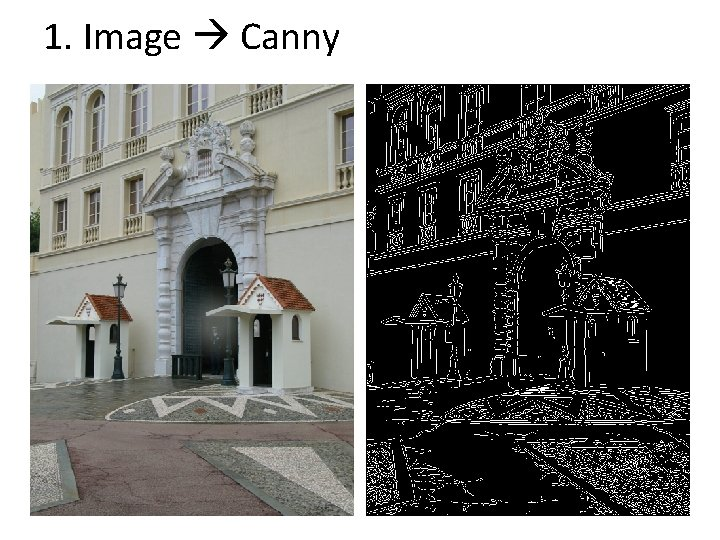1. Image Canny2. Canny lines … straight edges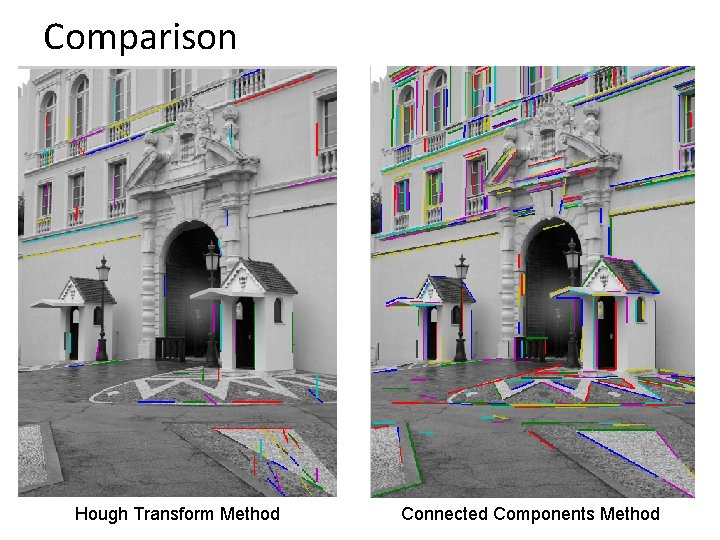Comparison Hough Transform Method Connected Components MethodThings to remember • Canny edge detector = smooth derivative thin threshold link • Generalized Hough transform = points vote for shape parameters • Straight line detector = canny + gradient orientations orientation binning linking check for straightness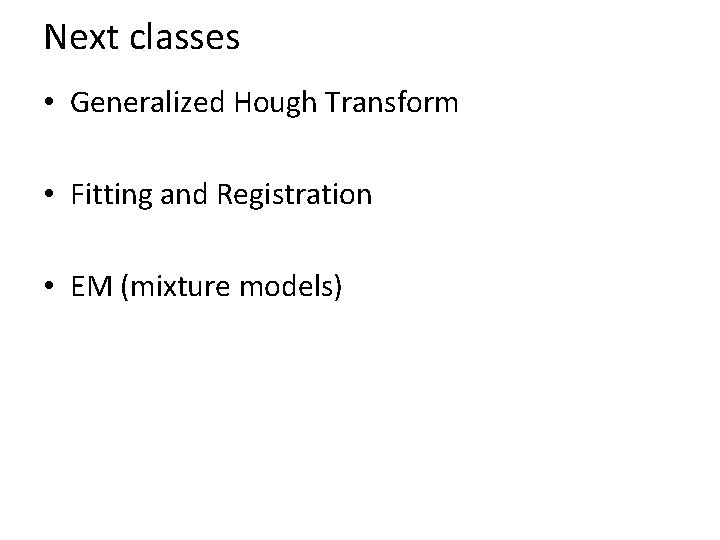Next classes • Generalized Hough Transform • Fitting and Registration • EM (mixture models)Questions